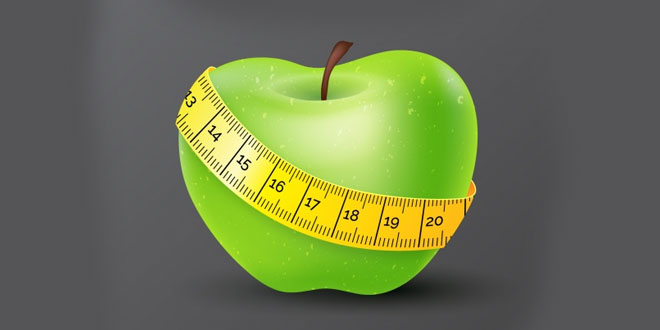Tuesday , October 27 2020# NCERT 5th Class (CBSE) Science: Measurement

#### Question: What is length?

Answer: The size or the measurement of something from one end to the other is called length.

#### Question: Name any two measures used in ancient times?

Answer: The two measures used in ancient times are:

1. Handspan
2. Cubit

#### Question: Why did people in ancient times realize the need for some fixed quantity of measurement?

Answer: The people in ancient times realize the need for some fixed quantity of measurement because the length of the various body parts differs from person to person.

#### Question: What is a unit?

Answer: A fixed quantity that is used as a standard of measurement is called a unit.

#### Question: Which unit would you use to measure the length of a pencil?

Answer: I would use cm (m) to measure the length of a pencil.

#### Question: Define temperature. Which instrument is used to measure it? Name the unit in which it is measured.

Answer: Temperature is the measure of how hot and cold an object is. It is measured using an instrument called a thermometer. Units used to measure temperature in degree Celsius (degree C) or degree Fahrenheit (degree F).

#### Question: Convert:

1. 2 hours into minutes – (60 min= 1 hr) 2*60=120 min.
2. 4 liters into milliliters – (1 ml = 1000 l) 4*1000 =4000 ml.
3. 20 minutes in seconds – (60 sec= 1 min) 20*60 = 1200 sec.
4. 10 meters into centimeters – (1 cm = 100 m) 10*100 = 1000 cm.
5. 20 kilograms to grams – (1 g = 1000 kg) 20*1000 = 20000 g.

#### Question: write the units for the following:

1. Finding out temperature of a patient having fever – Celsius (degree C)
2. Water in a big tank – Kilolitres (kl)/l.
3. Distance between your school and house -kilometre (km)
4. Water in a water bottle -Milliliter (ml)
5. Mass of the earth – kilogram (kg).

## स्वामी की दादी 5th NCERT CBSE Hindi Book Rimjhim Ch 13

स्वामी की दादी 5th Class NCERT CBSE Hindi Book Rimjhim Chapter 13 प्रश्न: “सच? राजम बड़ा बहादुर …

1.•